# KMP算法

## 串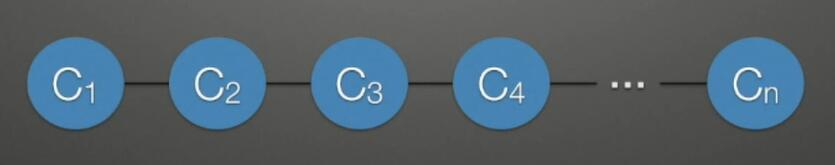``````// 串的定义
//typedef struct{
//    char str[maxSize+1];
//    int length;
//}Str;

// 或者
typedef struct{
char *ch;
int length;
}Str;``````

## 字符串匹配

### 简单模式匹配算法

``````// 简单模式匹配算法
// 假设字符串储存在1 ~ length上
int index(Str str, Str substr){
int i = 1, j = 1, k = i;
// 其中i和j分别用来表示主串和子串的位置, k用来暂存主串被比对的位置
while(i <= str.length && j <= substr.length){
if(str.ch[i] == substr.ch[j]){
++i;
++j;
}
else{
j = 1;
i = ++k; // 匹配失败 i从主串下一个位置开始
}
}
// 到这里有两种可能性 一个是原串被遍历完了 还有就是子串被遍历完了
// 如果是子串遍历完了说明匹配成功
if (j > substr.length)
return k;
else
return 0; // 因为假设字符串从下标1开始, 0是没有字符的
} ``````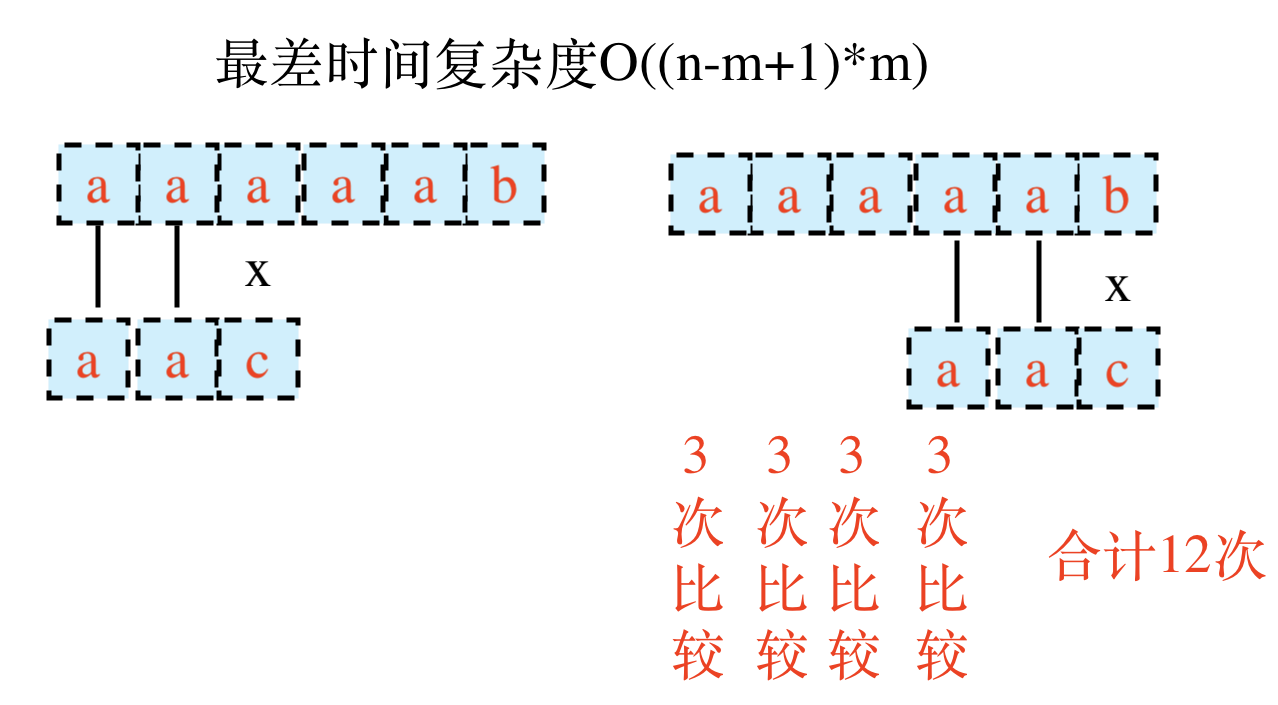### KMP算法

KMP算法是一种经典的字符串匹配算法, 相较于前面所说的简单字符串匹配算法, 在比较速度上有了相当大的提升.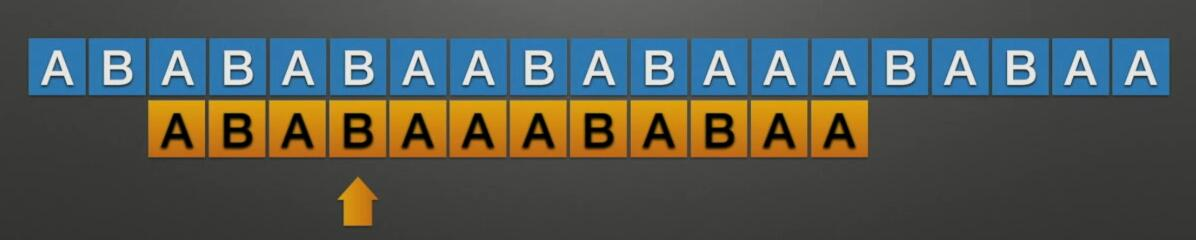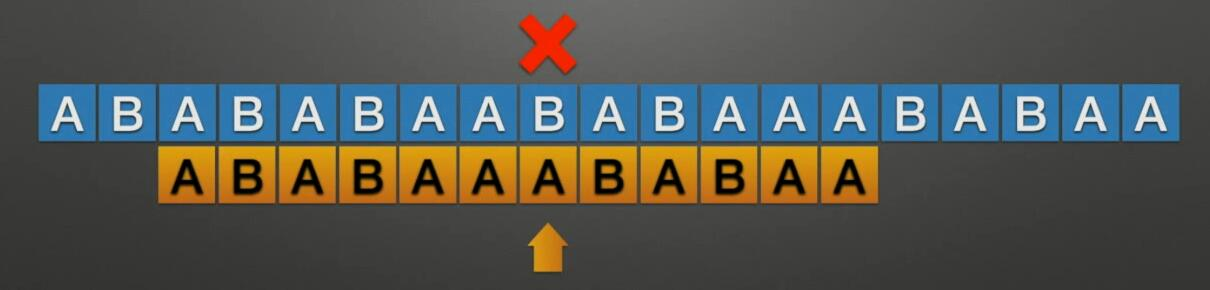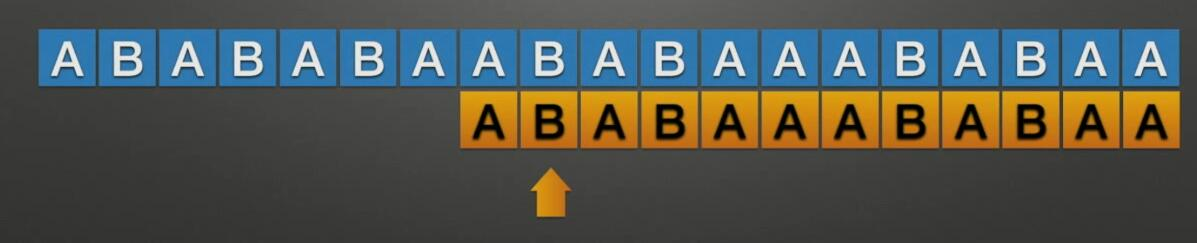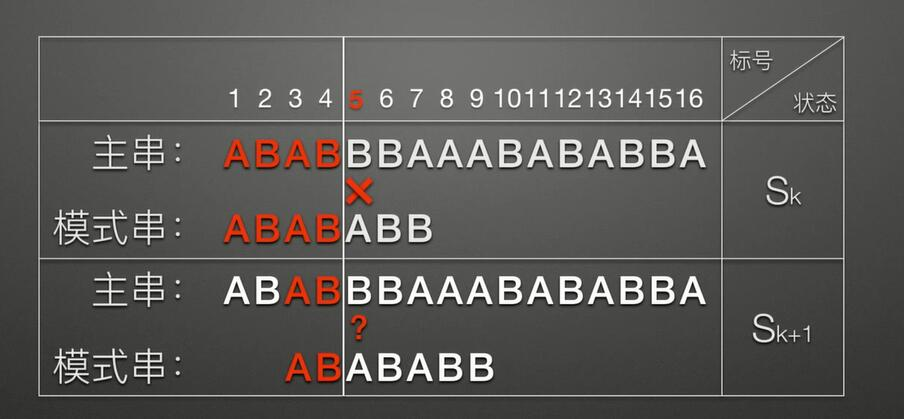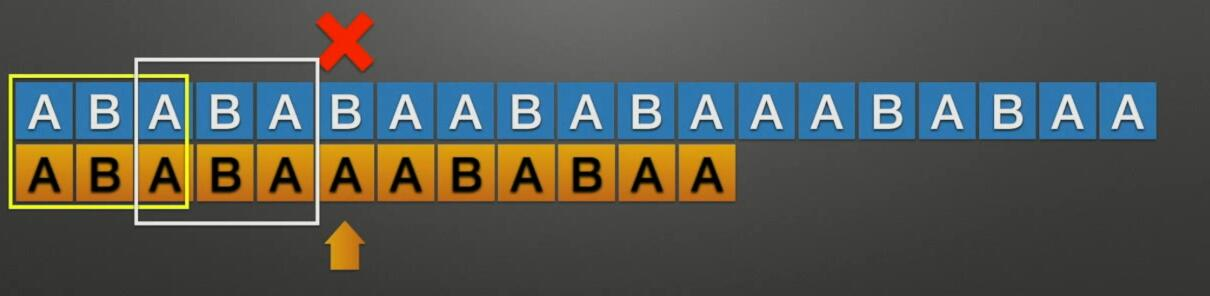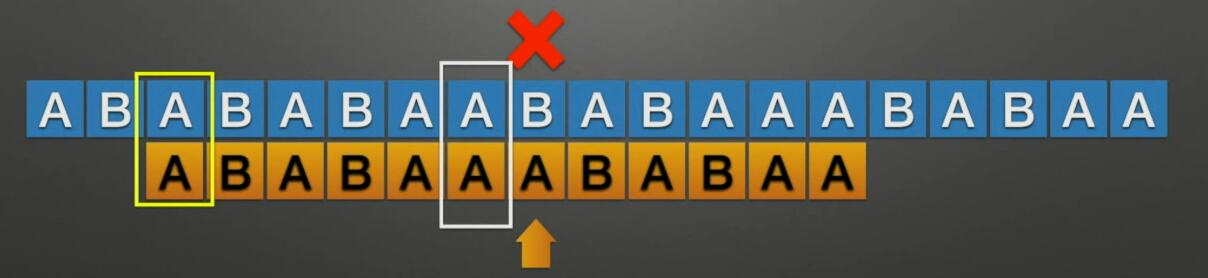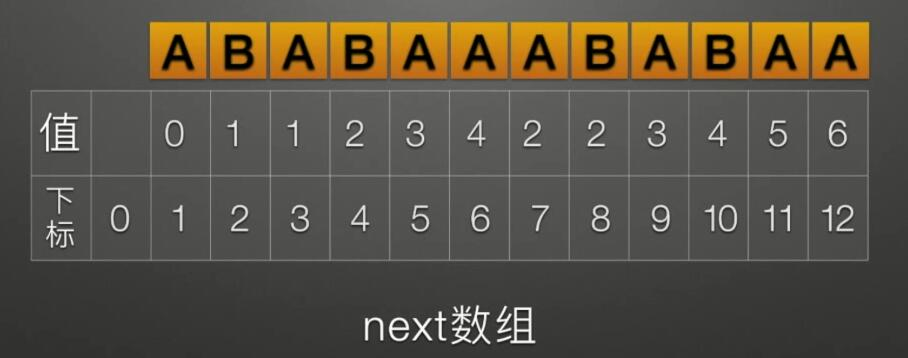100(特殊)-
201-
301-
412`A`
523`AB`
634`ABA`
712`A`
812`A`
923`AB`
1034`ABA`
1145`ABAB`
1256`ABABA`

#### 简单匹配升级到KMP

``````int KMP(Str str, Str substr, int next[]){
int i = 1, j = 1; // 下标从1开始
while(i < str.length && j < substr.length){
// 主串指针下移的情况
if(j == 0 || str.ch[i] == substr.ch[j]){
++i;
++j;
}
// 发生不匹配时模式串指针跳到next数组所指向的位置
else j = next[j];
}
// 遍历完模式串没发现不匹配 说明模式串与主串相匹配
if(j > substr.length) return i - substr.length;
else return 0;
}``````

#### 求next数组• 如果Pj = Pt, 那么next[j+1] = next[j]+1 = t+1.

• 如果Pj != Pt, 就得在这两个串(本质是模式串自己) 中找到最长的公共前后缀, 也就回到了字符串匹配的问题中. 将P(j-t+1) ~ Pj 视为主串, P1 ~ Pt视为模式串, 继续做字符串匹配. 必须向前反复重定位指针, 找到一个位置使得Pj = Pt或满足t = 0, 即将t循环赋值为next[t], t = 0 时, 令next[j+1] = 1.

注意, 因为第二种情况与字符串匹配完全一致, 所以建立next数组的代码一定与KMP算法极其相似:

``````// 求next数组的方法 substr为模式串
// j和t与上述图中相同
void getNext(Str substr, int next[]){
int j = 1, t = 0;
next = 0;
while(j < substr.length){ // j<= substr.length 会使next数组下标越界
// 模式串自身匹配
// t可能被下面的else赋值为0, 在条件并入后会将t置为1
// 并且第一次执行, 有next = 1, 满足之前推导的结果
if (t == 0 || substr.ch[j] == substr.ch[t]){
++j;
++t;
next[j] = t; // next[j] = length+1 length实际上就是没++前的t
}
else t = next[t]; // 模式串指针重定位到next[t]
}
} ``````

#### KMP算法改进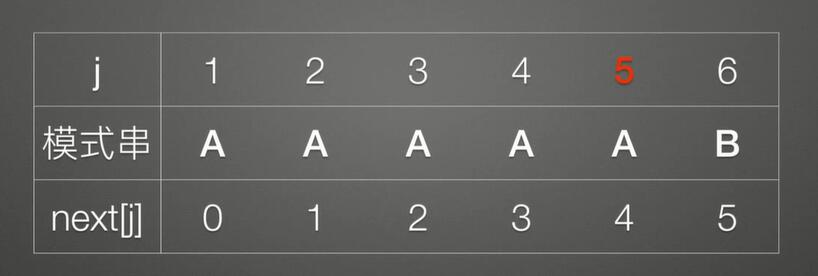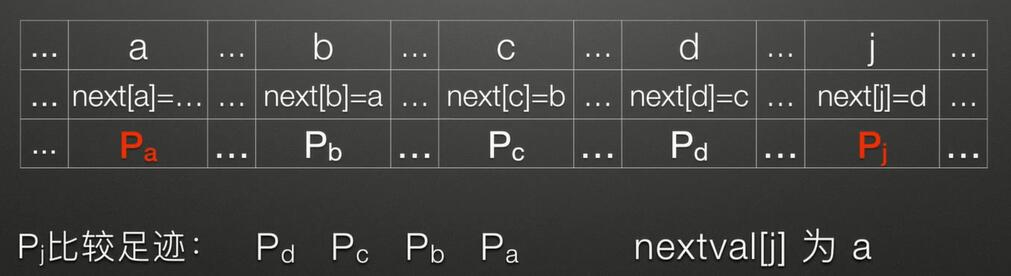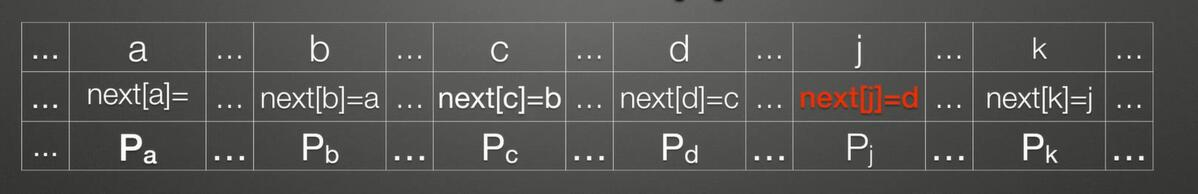1. j = 1时, nextval[j] 赋值为 0, 作为特殊标记
2. j > 1时:
• 若Pj != P(next[j]), 则nextval[j] = next[j].
• 若Pj = P(next[j]), 则nextval[j] = nextval[next[j]].

``````void getNextval(Str substr, int nextval[]){
int j = 1, t = 0;
nextval = 0; // j=1时nextval[j] = 0
while(j < substr.length){
if(t == 0 || substr.ch[j] == substr.ch[t]){
++j;
++t;
// 求解next数组时, 有next[j] = t; 那么t可以代替next[j]
if(substr.ch[j] != substr.ch[t])
nextval[j] = t; // nextval[j] = next[j]
else
nextval[j] = nextval[t]; // nextval[j] = nextval[next[j]]
}
else t = nextval[t];
}
}``````

上一篇别再对类别变量独热编码

2020-09-04Seq2Seq和Attention
2020.09.25: 本质部分的猜想被证实. 2020.09.21: 更新Attention的本质. 2020.09.19: 在接触了更多NLP内容后, 发现Attention是一个有特殊表征意义的结构, 以后会加入更深的理解. Se
2020-08-25
目录